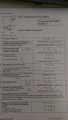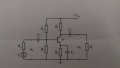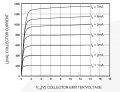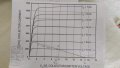# Common Emitter Amp Design help!!

#### FlyingRat

Joined Jan 26, 2016
14
Hello guys, this is my first post.
I have a project to design a common emitter amplifier. I was given only 2 information considering the whole circuit.
So I have to construct the whole amplifier which has Vcc=15V and transistor power (P=around 1W). I have to choose all the values (resistors, capacitors, type of a transistor,...) and make the whole calculation (DC,AC condition) including amplitude and phase characteristics. I don't have problems with calculation, but with designing. I was working with KSC2328A (NPN transistor). Is this the good one I have chosen?
So I found one webpage that helped me solving parts for DC conditions, and I will attach it for you to give me an opinion if I'm going in the right direction. Next step is to choose decoupling capacitors and I have a problem with that. I can't find any formulas considering capacitors, so I need Your help about that.
I will attach 2 pictures ("solved" DC conditions and schematic). Please help and tell me if I'm going in a good direction.#### thumb2

Joined Oct 4, 2015
122
The power dissipation of the transistor is approximated to:

$$P_D \approx V_{CE}I_C$$

If this is an homework it should be moved to the section "Homework help".
I can't check the calculus now.

#### FlyingRat

Joined Jan 26, 2016
14
Yes, I know the formula.
Thank You anyways. But I really don't know from which point should I start this. Should I start it from output characteristics of a transistor by drawing Q point and reading Ic, Ib, Vc-e or how I started with calculating resistors, voltages, currents, etc...?
I'm a new guy here, so should I delete the thread and repost in 'Homework help?' Or is there a shortcut to just move the thread?

#### FlyingRat

Joined Jan 26, 2016
14
EDIT: It says that I have to pick a point of a 'Q' along the load lines (AC & DC) for the maximum output voltage swing. So I guess I have to start planning from output characteristics of a transistor.Did I choose the right transistor? Power dissipation is 1000mW.
So what do you suggest me to do next? Should I start planning analytic way (like I did in the first post) or through output characteristics by drawing AC & DC load lines and selecting 'Q' point?
I have included output characteristics in the attachment.#### thumb2

Joined Oct 4, 2015
122
FlyingRat" said:
I choose the right transistor? Power dissipation is 1000mW.
I would choose a transistor with a little bit higher power dissipation. It is not a good idea to select components with maximum ratings exactly as the values given by the statement.

FlyingRat" said:
Should I start planning analytic way (like I did in the first post) or through output characteristics by drawing AC & DC load lines and selecting 'Q' point?
Yes, you are right. You should determine your Q (quiescent) point by graphical analysis, with a graph VCE vs IC as you attached.

If you have some doubt about Q point, it is explained here:
http://uotechnology.edu.iq/dep-eee/lectures/3rd/Electronic/Analog electronic/5.pdf

Once you have choose the Q point (VCE, IC and IB), we can proceed with component values.

#### WBahn

Joined Mar 31, 2012
29,163
Is the 1 W in the first post the power limitation on the transistor or the power that the amp is supposed to deliver to the load or what?

Do you have a specification for the impedance of the load?

#### FlyingRat

Joined Jan 26, 2016
14
Is the 1 W in the first post the power limitation on the transistor or the power that the amp is supposed to deliver to the load or what?

Do you have a specification for the impedance of the load?
Hey, thank You for the reply. I was told that "1 Watt" power is the power limitation (max.disipation of transistor), and for the load I was not given any specifical instructions. I asked my teacher and he said that it's the right way to start from output characteristics.
So I suppose I should draw AC & DC load lines that will give me 'Q' point. What's next?

#### dannyf

Joined Sep 13, 2015
2,197
transistor power (P=around 1W).
Not sure what that meant. It is unusual to design a SE amplifier outputting that much power so I suspect that it is the upper limit of the transistor's power dissipation.

A typical design process goes like this:

1) you want to maximize the swing of the output. So Vc = 1/2 Vcc = 7.5v;
2) you need to pick the Ic of the circuit. Depending on the load / application, from a few ma to maybe 10-20ma. Let's say 5ma here. Vc / Ic sets Rc;
3) once Rc is set, you can calculate Rc / gain = Re for the emitter resistor.
4) from Ic, you can estimate worst case Ib = Ic / beta (minimal). I would pick 50 or 100x as minimal beta for a small signal transistor.
5) based on that, you want the current flowing through the bias resistors to be at least 10x of the calculated Ib. So the lower resistor value Rb2 satisfies: (10*Ib)*Rb2 = 0.7v + Ic * Re
6) calculating the upper resistor value Rb1 is a child's play.

#### FlyingRat

Joined Jan 26, 2016
14
I would choose a transistor with a little bit higher power dissipation. It is not a good idea to select components with maximum ratings exactly as the values given by the statement.

Yes, you are right. You should determine your Q (quiescent) point by graphical analysis, with a graph VCE vs IC as you attached.

If you have some doubt about Q point, it is explained here:
http://uotechnology.edu.iq/dep-eee/lectures/3rd/Electronic/Analog electronic/5.pdf

Once you have choose the Q point (VCE, IC and IB), we can proceed with component values.
Ok, I determined my Q point for the maximum voltage output swing (Vceq = Vcc/2 = 7.5V) with DC load line. And got my values as it ''says'' in the attachment (Icq = 200mA; Ibq = 1mA; beta = hfe = 200).
What about AC load line, should I draw it now, or should I proceed next with resistor calculation? If so, can you simplify me those calculations with some formulas, I know it's basic ohms law, but I want to be sure, as You are the expert.

Not sure what that meant. It is unusual to design a SE amplifier outputting that much power so I suspect that it is the upper limit of the transistor's power dissipation.

A typical design process goes like this:

1) you want to maximize the swing of the output. So Vc = 1/2 Vcc = 7.5v;
2) you need to pick the Ic of the circuit. Depending on the load / application, from a few ma to maybe 10-20ma. Let's say 5ma here. Vc / Ic sets Rc;
3) once Rc is set, you can calculate Rc / gain = Re for the emitter resistor.
4) from Ic, you can estimate worst case Ib = Ic / beta (minimal). I would pick 50 or 100x as minimal beta for a small signal transistor.
5) based on that, you want the current flowing through the bias resistors to be at least 10x of the calculated Ib. So the lower resistor value Rb2 satisfies: (10*Ib)*Rb2 = 0.7v + Ic * Re
6) calculating the upper resistor value Rb1 is a child's play.
Hey dannyf,
I took Your advice considering 'maximizing the swing of the output (Vceq = 1/2 Vcc),but for the next step I CANNOT pick the Ic of the circuit so SMALL. If you look at output characteristics of a transistor I picked up (attachment), Q would go so low in the 'cutoff region' if I choose for example 5mA for the Ic.#### Jony130

Joined Feb 17, 2009
5,457
Hey, but notice that if Ic = 0.2A and Vce = 7.5V the power dissipation in transistor Ptot = 0.2A*7.5V = 1.5W is way too big for such a small BJT.
Q would go so low in the 'cutoff region' if I choose for example 5mA for the Ic.
No,

#### FlyingRat

Joined Jan 26, 2016
14
Hey, but notice that if Ic = 0.2A and Vce = 7.5V the power dissipation in transistor Ptot = 0.2A*7.5V = 1.5W is way too big for such a small BJT.

No,
Yes, you are right considering Ptot, missed that. But then, how could I really draw DC load line with the such small Ic. If you look closely at my last attachment (output characteristics), Ic scale goes from 0-1400 mA with steps of 200 mA.#### FlyingRat

Joined Jan 26, 2016
14
The output characteristics will look almost exactly the same. Only the current will scale down.

And in reality you don't need output characteristics to design a amplifier.
Thank You Jony130,
I've read some of Your comments and You seem to be a pro in ''transistor' area. But in one comment you said that You need to know Rload for construction BJT amp and for further calculations. And all I got is Pdissipation and Vcc. So I guess the only way for me is to start it from output characteristics?
My first task is to choose the transistor and calculate values of resistors/capacitors based on Q point, max.voltage swing and frequency characteristics. (So I guess I 'should' start from Q point to get max voltage swing)

#### Jony130

Joined Feb 17, 2009
5,457

#### FlyingRat

Joined Jan 26, 2016
14
http://213.114.136.42/_pdf/KS/KSC2328A.pdf (fig. 6)
P=1W means that the maximum dissipation through BJT can be 1W, NOT that it should be constantly 1W.

#### Jony130

Joined Feb 17, 2009
5,457

#### FlyingRat

Joined Jan 26, 2016
14
So from which point should I start then...output characteristics? I still don't know resistance of the load.
Can you give me some starting tips, calculations, please.

#### Jony130

Joined Feb 17, 2009
5,457
Pick some random number for Ic or Rc (RL) it doesn't matter much here.

#### FlyingRat

Joined Jan 26, 2016
14
Pick some random number for Ic or Rc (RL) it doesn't matter much here.
But still I should consider that amp should give max. output voltage swing, so I have to watch for Icq/Ibq relation to maintain max.swing?!
I think there should be a starting point besides picking numbers for components randomly...

#### Jony130

Joined Feb 17, 2009
5,457
But in your amplifier without external load the voltage swing has almost nothing to do with Icq/Ibq. So this is why you can pick Ic valuer randomly.
And to get max.swing ensure that Vce = 0.5Vcc.